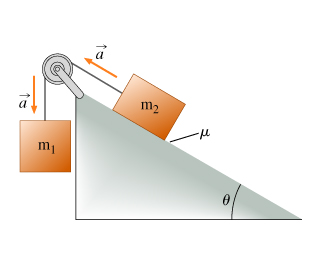# Problem: Block 1, of mass m1, is connected over an ideal (massless and frictionless) pully to block 2, of mass m2, as shown. Assume that the blocks accelerate as shown with an acceleration of magnitude a and that the coefficient of kinetic friction between block 2 and the plane is µFind the ratio of the masses m1/m2.Express your answer in terms of some or all of the variables a, µ, and θ, as well as the magnitude of the acceleration due to gravity g.

###### FREE Expert Solution

In this problem, we'll use Newton's second law of motion:

$\overline{){{\mathbf{F}}}_{\mathbf{n}\mathbf{e}\mathbf{t}}{\mathbf{=}}{\mathbf{m}}{\mathbf{a}}}$, where Fnet is the net force, m is mass, and a is acceleration.

The frictional force is expressed as:

$\overline{){\mathbf{f}}{\mathbf{=}}{\mathbf{\mu }}{\mathbf{N}}}$, where is the coefficient of friction and N is the normal force.

Weight:

$\overline{){\mathbit{w}}{\mathbf{=}}{\mathbit{m}}{\mathbit{g}}}$

The total force acting on m1 is:

m1g - T = m1a ................... (1)

86% (266 ratings)###### Problem Details

Block 1, of mass m1, is connected over an ideal (massless and frictionless) pully to block 2, of mass m2, as shown. Assume that the blocks accelerate as shown with an acceleration of magnitude a and that the coefficient of kinetic friction between block 2 and the plane is µ

Find the ratio of the masses m1/m2.
Express your answer in terms of some or all of the variables a, µ, and θ, as well as the magnitude of the acceleration due to gravity g.